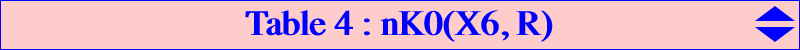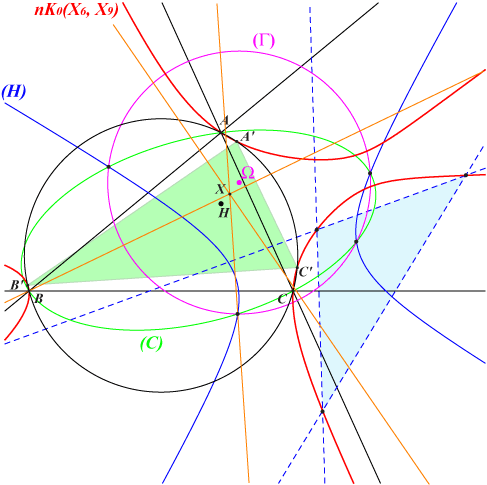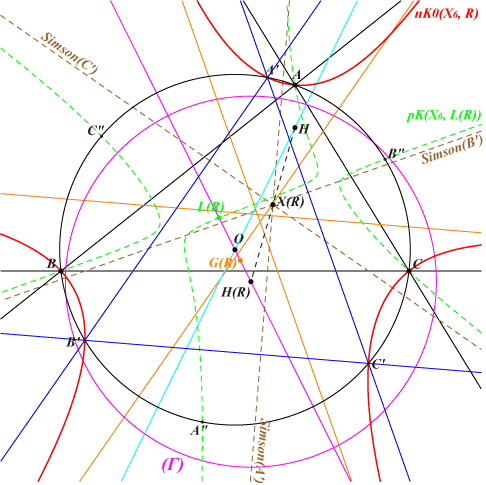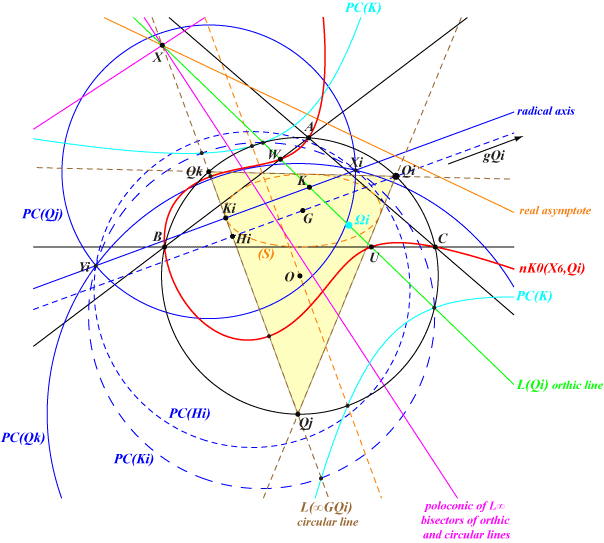The listed nK0(X6, R) are given in the following table.catalogue name R focal nodal K+ K60 circumconic K017 Brocard (first) X(385) K018 Brocard (second) X(523) YES Kiepert hyperbola K019 Brocard (third) X(647) YES Jerabek hyperbola K024 Kjp X(6) YES YES Circumcircle K040 Pelletier X(650) YES YES Feuerbach hyperbola K082 Equal power+ X(2) YES Steiner ellipse K137 Z+(IK) X(513) YES K164 van Lamoen X(2501) YES K188 Fermat focal X(1637) YES K189 Tarry focal X(230) YES K190 X(468) YES K221 X(661) YES K325 X(512)Given a point R = u:v:w (the root of the cubic), the isogonal nK0(X6, R) has barycentric equation : ∑ u x (c^2 y^2 + b^2 z^2) = 0. It always passes through the intercepts U, V, W of the trilinear polar of R on the sidelines of ABC. The points A and U, B and V, C and W share the same tangentials A', B', C' respectively which are three collinear points on the cubic. Any nK0(X6, R) can be considered as the locus of M such that M and its isogonal conjugate M* are conjugated with respect to : the circumconic (C) with perspector R, the diagonal rectangular hyperbola (H) passing through the in/excenters and whose axes are parallel to those of the circumconic above (when R ≠ X6), any conic of the pencil generated by these two conics, the circle (Γ) of this pencil. Any nK0(X6, R) can also be considered as the locus of M such that the circle with diameter MM* is orthogonal to (Γ). Note that the cubic contains the vertices of the diagonal triangle of the four basis points of the pencil although these are not necessarily all real. See figure below and also K082.The figure shows nK0(X6, X9) with some conics defined above. It is the locus of M such that M and its isogonal conjugate M* are conjugated with respect to any of the conics of the pencil generated by (C) and (H). This cubic meets the circumcircle again at A', B', C' whose Simson lines concur at X, the midpoint of HΩ, where Ω is the center of the circle (Γ) of the pencil. See H(R) below for further details.Special cubics Circular nK0(X6, R) nK0(X6, R) is circular if and only if R lies on the orthic axis. It is always a focal cubic with singular focus F on the circumcircle, isogonal conjugate of the point at infinity of the trilinear polar L of the isotomic conjugate of R. This line L passes through X(69) = isotomic conjugate of the orthocenter H. Such a cubic is the locus of foci of inscribed conics centered on a line passing through K called the axis or the orthic line of the cubic. This axis passes through the midpoints of AU, BV, CW. Hence the cubic always contains A, B, C, the circular points at infinity and the (four) foci of the in-conic with center K. Thus, all these cubics form a pencil of cubics. The general barycentric equation is : ∑ (-a^2 p + SB q + SC r) x (c^2 y^2 + b^2 z^2) = 0 where p:q:r is any point except the centroid G. Its singular focus is F = a^2(q-r) : : , its point at infinity is Inf = (q-r) : : , its real asymptote is the homothetic of the axis under h(F,2). See CL025. *** nK0+(X6, R) nK0(X6, R) has concurring asymptotes if and only if R lies on the cubic K138. This cubic contains X(2) giving K082 (Equal power+ cubic) and X(6) giving Kjp = K024. *** Equilateral nK0(X6, R) nK0(X6, R) is equilateral if and only if R is the Lemoine point K = X(6). This is Kjp = K024. *** Nodal nK0(X6, R) nK0(X6, R) is a nodal cubic if and only if it contains one and only one of the in/excenters which is therefore the node. If we take the incenter I = X(1), R must lie on the antiorthic axis (trilinear polar of I). The nodal cubics with node I form a pencil of cubics which contains only one focal cubic when R = X(650) (Pelletier point). It is the Pelletier strophoid K040. *** Central circular nK0(X6, R) nK0(X6, R) is a central circular cubic if and only if its center (and singular focus) is one of the three (always real) points where the Grebe cubic K102 meets the circumcircle. These are the vertices of the Grebe triangle. Further details in CL001.Circumcircle intercepts nK0(X6, R) meets the circumcircle (O) of ABC again at three points A', B', C' which are not necessarily real nor distinct. These are the vertices of a triangle denoted T(R). Here are a few special cases : • When nK0(X6, R) is circular, T(R) has vertices the singular focus F and the circular points at infinity. This case is excluded in the sequel. • When R = X(6), nK0(X6, R) = K024 is equilateral, T(R) is the CircumTangential triangle. • When R = X(2), nK0(X6, R) = K082, T(R) is ABC itself. *** Centroid G(R) of T(R) Back to the general case, the centroid of T(R) is the point G(R) = (–3 a^2 + b^2 + c^2) u + 2 a^2 (v + w) : : . Clearly, G(X2) = X2 and G(X6) = X3. Other examples are given in ETC, see X(35258)-X(35306). G(R) lies on the following lines : {X6, X1992 x R}, {X6 x cR, X193 x R}, {X6 x aR, X69 x R}, {X6 x ∞GR, R}. More generally, let Rt and Tt be the points defined by GRt = t GR and GTt = 2 / (1+t) GK (vectors) then G(R) lies on the line passing through the barycentric products X6 x Rt and R x Tt. When t varies, the line RtTt envelopes a hyperbola with center cR, passing through X1992 with tangent passing through G, with asymptotes passing through R and X193. Some properties : • The fixed points of R -> G(R) are X(2), X(5638), X(5639). The two latter points lie on the Lemoine axis which is therefore globally invariant. The other lines are the axes of the Steiner ellipse which are also globally invariant. • When R lies on the Euler line, G(R) lies on the line passing through X2 and X154. • When R lies on the line passing through X2 and X6, G(R) lies on the Euler line. • More generally, when R lies on a line passing through X2, G(R) lies on another line passing through X2. • When R lies on the circumconic with perspector X512, passing through X2 and X6, G(R) lies on the Jerabek-Thomson hyperbola (JT). It is the second point of (JT) on the line passing through X(6) and R. Note that these two conics meet at X(2), X(6), X(5638), X(5639). *** Orthocenter H(R) of T(R) H(R) is obviously the image of G(R) under the homothety h(X3, 3). It is the center of the circle (Γ) of the pencil mentioned at the top of the page. *** De Longchamps point L(R) of T(R) L(R) is obviously the reflection of H(R) in X3. Note that the antipodes A", B", C" of A', B', C' in (O) lie on pK(X6, L(R)). *** Simson lines The Simson lines of A', B', C' concur at X(R) which is the midpoint of H = X(4) and H(R). These Simson lines are the parallels at X(R) to the altitudes of T(R), also the perpendiculars at X(R) to the asymptotes of the cubic.Stuyvaert point of nK0(X6, R) In general, there is one and only one point S whose polar conic in nK0(X6, R) is a circle. This point is called the Stuyvaert point of the cubic. See Table 47. The mapping f : R -> S has three singular points namely the vertices of the Thomson triangle. In this case, nK0(X6, R) has a pencil of circular polar conics. See figure and further details below. f is a quadratic involution given by {u : v : w} -> a^2(c^2 SB v^2 + b^2 SC w^2 + SB SC v w + b^2 c^2 u (-u+v+w)) : : . f fixes the cubic K138, an equilateral cubic passing through X{2, 6, 5652, 14898, 14899}, the locus of roots R of isogonal nK0+ cubics i.e. nK0(X6, R) cubics with concurring asymptotes (at S). When R lies on K138, this point S also lies on K138 hence the mapping f : R -> S is an involution and a conjugation on K138. f maps the orthic axis (in which case the cubic is circular) to the circumcircle (O) and here, S is the singular focus of the cubic. f maps the trilinear polar of X(99) – i.e. the line X(2)X(6) – to the Jerabek-Thomson hyperbola which contains the vertices of the Thomson triangle. Remark : for a given point M, the points M, R, S are collinear if and only if R lies on a circumcubic of the Thomson triangle. With M = X(2), we obtain K1093 which is the only circumcubic of ABC of this type. ***L(M) is the trilinear polar of M in ABC and PC(M) is the polar conic of M in the cubic. The Thomson triangle is here denoted by QiQjQk. Since Qi lies on (O), nK0(X6, Qi) meets the sidelines of ABC on the line L(Qi) which must contain K. The polar conic of every point on the line QjQk with respect to the cubic nK0(X6, Qi) is a circle centered on QjQk and all these circles are in a same pencil. The cubic nK0(X6, Qi) is a K+ with only one real asymptote and the three asymptotes concur at X on QjQk. The polar conic of X splits into the line at infinity and the radical axis of the pencil. This is the parallel at K to the line GQi, meeting QjQk at Ki on the Steiner inscribed ellipse (S). The infinite point gQi of the line GQi obviously lies on the Thomson cubic. Its polar conic splits into the orthic line L(Qi) and the circular line L(∞GQi) = QjQk. Hence the tangents to the cubic at their six common points are parallel to the radical axis and perpendicular to QjQk. The polar conic PC(K) is a rectangular hyperbola with center Ωi on the Brocard circle, the orthogonal projection of O on L(Qi). See the related cubic K1141. PC(K) meets (O) on L(Qi) and on the parallel at O to QjQk. PC(K) also meets PC(Ki) on L(Qi) and two other points on QjQk.